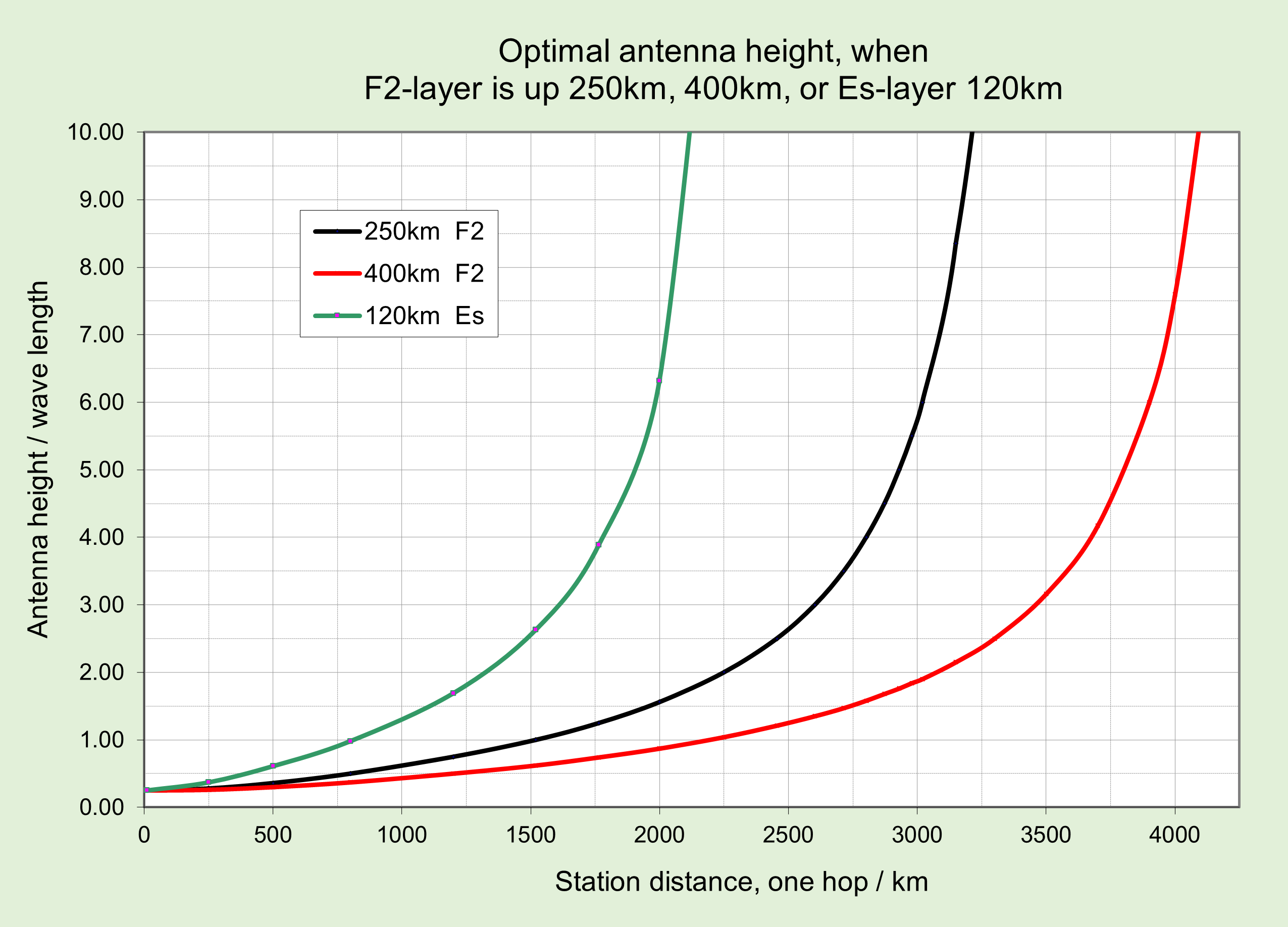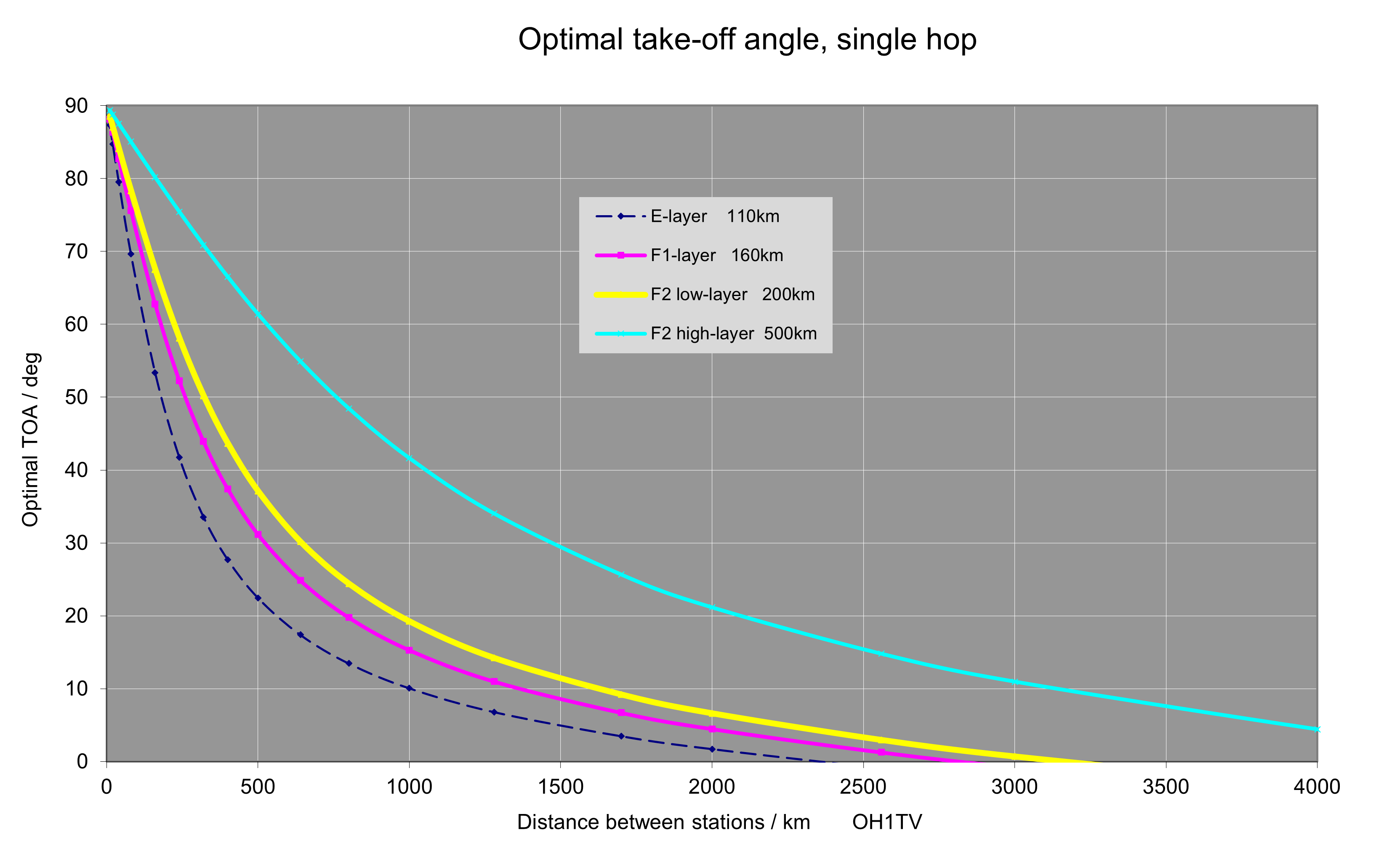### Optimal Take-Off Angle and Maximum Usable Frequency -calculator

This project was originally done in 2012 by using Excel as a calculator. Now in 2021 I have added a html + Java script version of it here. Link to the original Excel version, including the geometry model, is in the end of this page.

With this calculator you can estimate how well your antenna TOA and height are in line with the DX-distance in current conditions. Density and height of the reflecting layers vary. That information is available in Ionograms. As an example is current Ionogram from Juliusruh Germany.   Please note that best TOA does't depend on frequency, but antenna height does.

#### Calculator

Give station distance in km: , max 3000km, one hop, first hop.

Give height of the reflecting layer in km: , hmF2 or hmE from Ionogram.

Best take-off-angle is: ,     Best antenna height is:

MUF-multiplier is:

Give from Ionogram foF2 /MHz: . This is not needed for angle calculation.

Ionograms include also MUF-info. Readings there are often 10-20% lower. Their calculations use more complex algorithm. You can compare these to the real band situation. By using the calculator above you can calculate any combination.

The calculator can be used also for E-layer analysis. 40m conditions often happen via E-layer, at shorter distance. In this case use hmE and foE data.

Below is an example of how optimal antenna height varies as function of station distance and height of reflecting layer.Pekka Ketonen 11.6.2021